Reach Us+44-1522-440391
An Appropriate Extreme Value Distribution for the Annual Extreme Gust Winds Speed | OMICS International
Journal of Fundamentals of Renewable Energy and Applications
All submissions of the EM system will be redirected to Online Manuscript Submission System. Authors are requested to submit articles directly to Online Manuscript Submission System of respective journal.

# An Appropriate Extreme Value Distribution for the Annual Extreme Gust Winds Speed

Banafsheh Abolpour1, Bahador Abolpour2,3,4*, Hosein Bakhshi5 and Mohsen Yaghobi2

1Department of Civil Engineering, Science and Research Branch, Islamic Azad University, Iran

2Department of Chemical Engineering, Shahid Bahonar University of Kerman, Iran

3Department of Aerospace Engineering, Payame Noor University, Iran

4Department of Computer Engineering, Payame Noor University, Iran

5Department of Civil Engineering, Hakim Sabzevari University, Iran

*Corresponding Author:
Department of Chemical Engineering
Faculty of Engineering, ShahidBahonar University of Kerman
Post Code 76175, Kerman, Iran
Tel: 98-711-8312254
Fax: 98-711-8203239
E-mail: [email protected]

Received Date: December 08, 2016; Accepted Date: January 23, 2017; Published Date: January 25, 2017

Citation: Abolpour B, Abolpour B, Bakhshi H, Yaghobi M (2017) An Appropriate Extreme Value Distribution for the Annual Extreme Gust Winds Speed. J Fundam Renewable Energy Appl 7: 223. doi:10.4172/20904541.1000223

Copyright: © 2017 Abolpour B, et al. This is an open-access article distributed under the terms of the Creative Commons Attribution License, which permits unrestricted use, distribution, and reproduction in any medium, provided the original author and source are credited.

Visit for more related articles at Journal of Fundamentals of Renewable Energy and Applications

#### Abstract

In this study, an extreme value distribution of the gust wind speeds is obtained in a large selected area of Iran. The generalized Pareto distribution is used to find out the type of wind speed distribution. The three parameters of the generalized extreme value distribution function are reduced to either type I Gumbel, type II Frechet or type III reverse Weibull distribution function for the annual extreme gust wind speeds. It is obtained that, the annual extreme gust wind speeds at 102 stations have a reverse Weibull function distribution. It is also obtained that, type I Gumbel extreme value function is the best model for many of the studied stations.

#### Keywords

Annual extreme gust wind; Extreme value distribution; Gumbel; Frechet; Reverse Weibull

#### Introduction

Today, wind power is an important dynamically energy source. Using the wind as a source of energy has been increased . A set of wind turbines in a same location products the electrical power. To maximize this generated power, these locations are built far from shores or in open fields far away from buildings and trees. The suitability of these locations must be obtained using a set of data that approves the wind speed and direction in these locations.

It is observed that, extreme wind speeds are physically bounded. Weibull and reverse Weibull distributions are used for annual the extreme winds [2-5]. It is indicated that, annual fastest-mile wind speeds have the reverse Weibull distribution. A two-parameter generalized Pareto distribution is usable for analyzing the extreme gust wind speeds instead of all Type I distributions. The solution range of the tail-length parameter c of the generalized Pareto distribution may indicates the extreme events for c value approaching zero the Type I Gumbel, for c>0 the Type II Frechet and for c<0 the Type III reverse Weibull are suitable extreme value functions. More details have been presented in a previous study in USA .

Wind load on a structure is a function of various parameters such as wind speed and direction, geometry of the structure and local topography [7,8]. First, design and wind pressures on the external surfaces of the structure are calculated. Then these pressures are converted to the load impact. The amount of wind pressures on the structures in Iran is calculated using the following equations :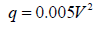(1)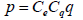(2)

Where p and q are the design and wind pressures (dN/m2), respectively. V is the basic wind speed (km/h) and Cq and Ce are the shape and speed variation coefficients, respectively. Analyzing the wind speed data indicates that, the maximum annual wind speed at particular locations follows Type I extreme value distribution. This type is the most frequently used model for wind speed [2,10]. The probability distribution of the wind load (as a function of V) is a valuable parameter for the structural design of buildings. Nevertheless, this parameter is not necessarily for Type I distribution, because the wind pressure is a function of V2 (instead of V). Since some of parameters are random in nature, it is difficult to determine the wind load distribution. Previous studies showed the uncertainty of the wind load and low values of c in the cumulative distribution function (CDF), consequently. Therefore, wind load can be represented by a Type I distribution [2,4,5,11] for close to zero values of c.

Procedure

Considering W (t) as a series of wind speed at a specific site and V as the extreme gusts associated with W (t), the generation of V distribution is not known. However, V can be estimated using its tail quantile probability of a suggestion contingent cumulative distribution function (CDF), F(v), (for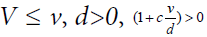and V=W (t) - u where u is a sufficiently large threshold of W (t)):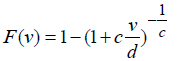(3)

Where v is a contingent investigation of V and c and d are the shape and scale parameters, respectively. Equation 3 is the generalized pareto distribution (GPD) and will considered an extreme gust of wind as the basis for the review. Element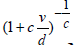on the right side of this Equation is known as the generalized Pareto. Many researchers used the GPD to estimate annual wind speed extreme [4,5,11,12].

#### Generalized Distribution of Values

Wide distribution type I (Gumbel)

There is strong evidence in scientific literature, which advocates the use of the Gumbel distributions to fit extremes events [13-18]. Wide distribution of values as its name implies is useful to describe the probabilistic nature . CDF of this variable for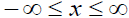is: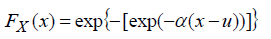(4)

Where u and α are parameters of the distribution. The mean and standard deviation can be calculated using the following limits: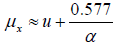(5)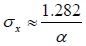(6)

Therefore, if the mean and standard deviation are determined, equations5 and 6 can be changed and the corresponding values for the distribution parameters can be obtained from these equations.

Wide distribution type II

Sometimes the best estimate of the distribution of the maximum load on a structure can be provided by Type II [20,21]. CDF of this variable for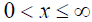is: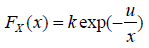(7)

Where u and k are parameters of the distributionand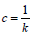for c>0. The mean and standard deviation can be calculated using the following limits: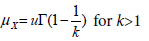(8)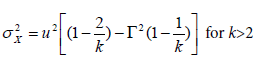(9)

Note that, the coefficient of variation, Vx, is a function of k and is calculated elsewhere .

Wide distribution type III (Weibull)

This distribution is defined by three parameters. There are different functions for the largest and smallest values . For x ≤ w CDF can be defined as follow: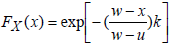(10)

Where w, u and k are parameters of the distribution and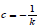for c<0. Mean and variance also are calculated using the following equations: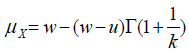(11)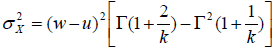(12)

For x ≥ Æ� CDF is defined as follow: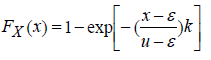(13)

Where Æ�, u and k are parameters of the distribution. Mean and variance for this range of values is defined as follows: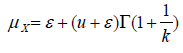(14)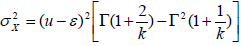(15)

Gamma distribution is defined as follow: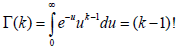(16)

If the GPD assumption were correct, the plot of the cumulative mean exceedance (CME) should follow a straight line. Therefore, c and d can be obtained from the characteristics of this line.

#### Data Preparation

Wind speed data was obtained from the center of Iran weather databases. This database included 287 weather stations. 181 of these stations had data ranged from 5 to 54 years. It was felt that hurricanes and tornadoes were worthy of separate consideration. Thus, the stations had been affected by hurricanes and tornadoes were deleted. A minimum record length of 15 years was chosen to allow an adequate amount of wind data to be analyzed in this study. Because of climatic characteristics, a smaller sample may not adequately represent all possible wind patterns. Therefore, stations with less than 15 years of record were removed from the database. Finally, 109 meteorological stations were selected for analysis.

#### Evaluate the Information and Forms

Figures 1 and 2 show the mean, standard deviation and maximum values of the annual extreme gust wind speed at all 109 stations in Iran. The parameters c and d were quantified using the CME method. The annual series of median wind speed, Vmed, provided satisfactory results . It is assumed that the extreme events occur in succession.

Figure 1: Annual gust wind mean speeds and standard deviation of these values at 109 meteorological stations of Iran.

Figure 2: Maximum values of annual gust wind speeds at 109 meteorological stations of Iran.

As a result, pieces of nonlinear CME can be removed. Thus the parameter c will obtain with a more accurate. These findings, is identical with the results by other researchers [23,24]. The extreme gust wind v is plotted against the reduced variate W, by both Gumbel and reverse Weibull distributions in Figures 3-5.

Figure 3: Gumbel and reverse Weibull distribution applied for annual extreme gust wind speeds in Kerman, Rafsanjan station (1973-1987).

Figure 4: Gumbel and reverse Weibull distribution applied for annual extreme gust wind speeds in Kerman, Bam station (1975-1990).

Figure 5: Gumbel and reverse Weibull distribution applied for annual extreme gust wind speeds in Kerman, Sirjan station (1978-1992).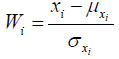(17)

As shown in Figures 6 and 7, Type III reverse Weibull distribution, are most suitable for delineating the annual extreme gust wind speeds at the 109 stations; So the inverse Weibull distribution for 109 stations, is the basic representative. The result is in agreement with the conclusion suggested by other studies for severe winds. However, analysis of data of VRN (wind speed estimates using the reverse Weibull distribution) and VGN (wind speed estimates using the Gumbel distribution) present in these figures show the opposite results. It is observed that VRN and VGN, for gust winds with great intervals in many of stations, Type I Gumbel distribution has a higher accuracy than Type III distribution. The results of about 102 stations for both Types I and III distributions are approved. Some examples in Figures 3-5 are presented.

Figure 6: Estimated gust wind speed estimates based on reverse Weibull distribution at 109 meteorological stations of Iran.

Figure 7: Estimated gust wind speed estimates based on Gumbel distribution at 109 meteorological stations of Iran.

#### Conclusion

Preliminary annual extreme gust wind speed distribution in the selected 109 stations in Iran was investigated. Based on calculations using CME, it is obtained that the annual extreme gust wind speeds at 102 stations have a reverse Weibull function distribution. However, the results of data analysis and graphic curves showed that Type I Gumbel extreme value function is the best model for many of the studied stations. Wind speed predictions based on Gumble distribution may be unsuitable for the most of time intervals. However, taking into account the time intervals, for a maximum duration of 54 years in 109 selected stations, distributed type I in the modeling extreme gust wind speed, at 102 stations have represented better results.

#### References

Select your language of interest to view the total content in your interested language

### Article Usage

• Total views: 1586
• [From(publication date):
January-2017 - Jul 19, 2019]
• Breakdown by view type
• HTML page views : 1436Can't read the image? click here to refresh